# Best Probability and Statistics Books for Engineers and Self Learners

Probability and Statistics are two branches of mathematics where probability deals with the measure of the likelihood that an event will occur in a random experiment and statistics deal with the collection, analysis, interpretation, presentation, and organization of data. Here you will get the best probability and statistics books

## 1. Student's Solutions Guide for Introduction to Probability, Statistics, and Random Processes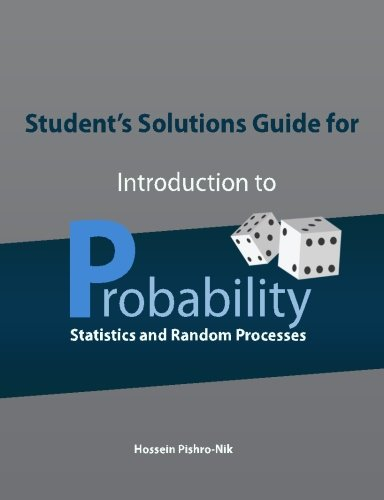Author: Hossein Pishro-Nik
Published at: 20/06/2016
ISBN: 0990637212

A very practical and useful book for both first-time learners and self-learners which includes practice problems at the end of each section, very helpful and easy to understand examples, easy to follow and complete solutions, the book webpage which provides examples both in MATLAB and R.

Student's Solutions Guide for Introduction to Probability, Statistics, and Random Processes explains the following topics very clearly

• Continuous density functions
• Moment generating functions
• Probability bounds
• Random experiments
• Probability axioms
• Conditional probability
• Counting methods
• Single and multiple random variables
• Characteristic functions, random vectors, and inequalities
• Limit theorems and convergence
• Introduction to mathematical statistics
• Processing of random signals
• Poisson processes
• Discrete-time and continuous-time Markov chains
• Brownian motion
• Simulation using MATLAB and R

This book is a very good fit for an undergraduate-level textbook on probability with well-explained steps to help students better understand the subject and learn the necessary techniques to solve the problems.

## 2. Statistics Laminate Reference Chart: Parameters, Variables, Intervals, Proportions (Quickstudy: Academic)

An excellent reference for novice learners and for anyone studying statistics or AP statistics. These training aids help you pass business, finance, and economics courses and educational information such as formulas and theories are explained in an easy to understand manner.

You'll learn

• EPPP
• Algebra and Word Problems
• How to locate formulas, without flipping through the textbook, or trying to memorize them all.
• Z and T tables

You'll get some

• Basic definitions
• Frequency distribution
• Measures of central tendency
• Measures of dispersion
• Graphing techniques

These pamphlets have comprehensive information and cover a wide range of course outlines with a great explanation.

## 3. Probability and Statistics for Engineering and the Sciences

Probability and Statistics for Engineering and the Sciences is a decent introduction to statistics and covers quite a few topics relatively quickly by providing output, graphics, and screenshots from various statistical software packages. Jay L. Devore emphasizes authentic problem scenarios in a multitude of examples and exercises, many of which involve real data, to show how statistics make sense of the world.

Contents include

• Overview and descriptive statistics
• Probability
• Discrete random variables and probability distributions
• Continuous random variables and probability distributions
• Joint probability distributions and random samples
• Point estimation
• Statistical intervals based on a single sample
• Tests of hypotheses based on a single sample
• Inferences based on two samples
• The analysis of variance
• Multifactor analysis of variance
• Simple linear regression and correlation
• Nonlinear and multiple regression
• Goodness-of-fit tests and categorical data analysis
• Distribution-free procedures
• Quality control methods.

## 4. Probability and Statistics (4th Edition)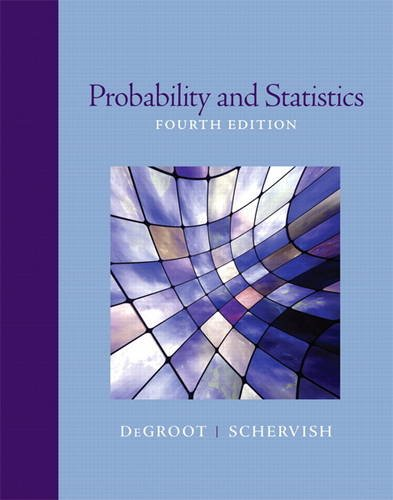Author: Morris H. DeGroot,Mark J. Schervish
Published at: 06/01/2011
ISBN: 0321500466

Probability and Statistics are for master level and higher year undergraduate students. It provides clear examples and exercises with "additional questions" at the end of each chapter which really helps improve learning and there is a logical progression from one idea to another.

Coverage includes

• Introduction to probability
• Conditional probability
• Random variables and distributions
• Expectation
• Special Distributions
• Large random samples
• Estimation
• Sampling distributions of estimators
• Testing hypotheses
• Categorical data and nonparametric methods
• Linear statistical models
• Simulation.

## 5. Introduction to Probability and Statistics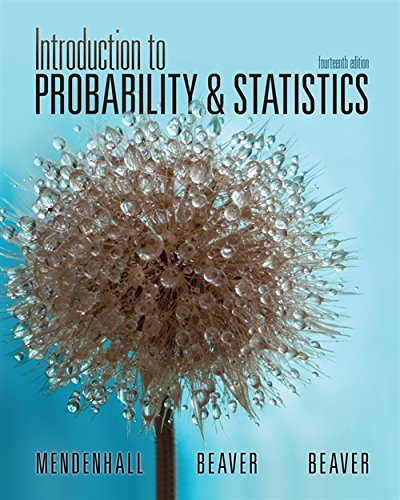Author: William Mendenhall,Robert J. Beaver,Barbara M. Beaver
Published at: 01/01/2012
ISBN: 1133103758

Introduction to Probability and Statistics is a very nice summary of key points and calculations. By reading this book you will gain a solid understanding of statistical concepts

What you will learn

• How to apply statistical procedures
• How to describe real sets of data
• What statistical tests mean in terms of practical application
• How to evaluate the validity of the assumptions behind statistical tests
• What to do when statistical assumptions have been violated
• How to apply tools in the real world.

Important topics are

• Describing data with graphs
• Describing data with numerical measures
• Describing bivariate data
• Probability and probability distributions
• Several useful discrete distributions
• The normal probability distribution
• Sampling Distributions
• Large-sample estimation
• Large-sample tests of hypotheses
• The inference from small samples
• The analysis of variance
• Linear regression and correlation
• Multiple regression analysis
• Analysis of categorical data
• Nonparametric statistics.

## 6. Schaum's Outline of Probability and Statistics, 4th Edition: 897 Solved Problems + 20 Videos (Schaum's Outlines)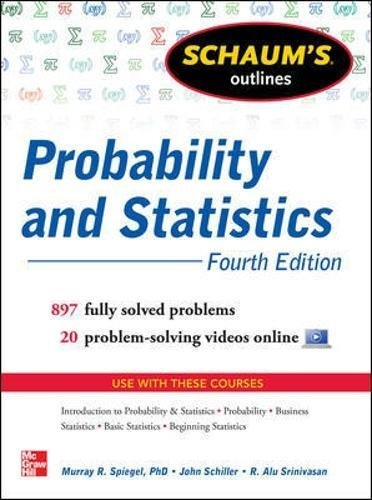Author: John J. Schiller Jr.,R. Alu Srinivasan,Murray R. Spiegel
Published at: 06/12/2012
ISBN: 007179557X

Schaum's Outline of Probability and Statistics gives you great exercises and solved problems, real-life examples like games or rial historic events, necessary steps to compute statistical or probability outputs from which you'll find everything that you need to build confidence, skills, and knowledge for the highest score possible.

This book provides you

• More than 750 fully solved problems
• Examples
• Practice exercises to sharpen your problem-solving skills

Important coverage are

• Basic probability
• Random variables and probability distributions
• Mathematical Expectation
• Special probability distributions
• Sampling theory
• Estimation theory
• Tests of hypotheses and significance
• Curve fitting, regression, and correlation
• Analysis of variance
• Nonparametric tests
• Bayesian methods.

## 7. Probability, Statistics, and Decision for Civil Engineers (Dover Books on Engineering)Author: Jack R Benjamin PhD,C. Allin Cornell PhD
Published at: 16/07/2014
ISBN: 0486780724

Probability, Statistics, and Decision for Civil Engineers is an amazing work on civil engineering epistemology which is designed as a primary text for civil engineering courses. It is a self-study by practicing engineers and also designed as a supplementary text for courses in other areas. This is very useful as a reference text.

What you'll get

• Extensive use of examples and illustrations will help you develop an in-depth appreciation for the theory's applications.
• Fundamental and valuable discussions for conceptual understandings of P&S
• Risk and uncertainty
• How to make a decision under uncertainty
• Conceptual and mathematical insights
• Bayesian statistical decision theory
• subjective probability, and utility theory
• Analyzing engineering economic decisions in the face of uncertainty
• An Appendix of tables.

## 8. Probability & Statistics with Applications: A Problem Solving Text, 2nd Edition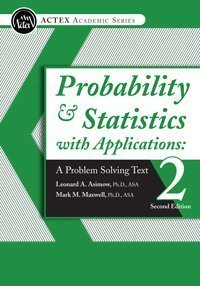Author: ASA Leonard A. Asimow Ph.D.
Published at: 01/01/2015
ISBN: 1625424728

Probability & Statistics with Applications is one of the most helpful and well-written textbooks to learn probability and statistics. This book covers all the topics that are relevant to SOA Exam C and is a must-read supplement for those who are preparing for the P Exam for an actuary.

This book provides you

• An abundance of illustrative examples
• 870 exercises
• Mathematical probability and statistics
• Discrete and continuous mixture distributions
• Non-homogeneous Poisson processes
• Conjugate pairs in a Bayesian estimation
• Statistical sufficiency
• Non-parametric statistics.

## 9. Introduction to Probability (Chapman & Hall/CRC Texts in Statistical Science)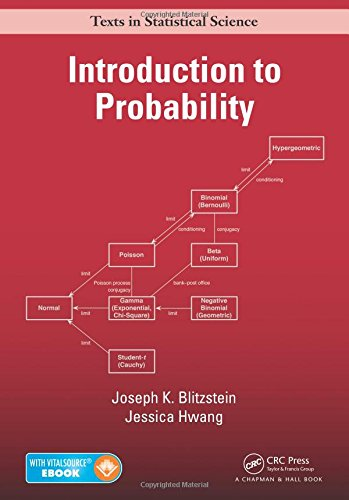Author: Joseph K. Blitzstein,Jessica Hwang
Published at: 24/07/2014
ISBN: 1466575573

This book provides essential language and tools for understanding statistics, randomness, uncertainty, explores a wide variety of applications and examples, provides information about additional application areas include genetics, medicine, computer science, and information theory. In this book, the author shows how to perform relevant simulations and calculations in R.

Important topics are

• Probability and counting
• Conditional probability
• Random variables and their distributions
• Expectation
• Continuous random variables
• Moments
• Joint Distributions
• Transformations
• Conditional expectation
• Inequalities and limit theorems
• Markov chains
• Markov chain Monte Carlo
• Poisson processes
• Math and R

## 10. CliffsNotes Statistics and Probability Common Core Quick Review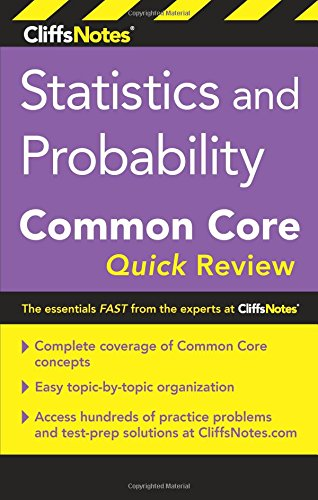Author: Malihe Alikhani M.S.
Published at: 14/02/2017
ISBN: 0544734122
In this book, Malihe Alikhani M.S. provides statistics and probability common core math standards, practice problems, chapter-end quizzes, and 300+ multiple-choice questions.

Key contents are

• Overview of statistics
• Interpreting graphics displays
• Measures of central tendency and variability
• Probability
• Random sampling
• Principles of hypothesis testing
• Univariate inferential tests
• Bivariate relationships.

Four tables at the end of the book on

• Binomial probabilities, P(x) for n < 20
• Standard normal probabilities
• The t-distribution critical values
• The chi-square distribution: X2 critical values.

Thanks for reading this post. If you have any opinion don't hesitate to comment here. Also please subscribe our newsletter to get more updates.